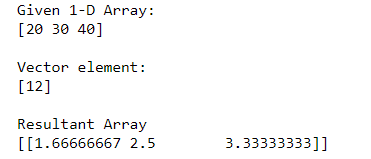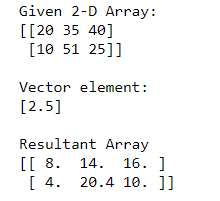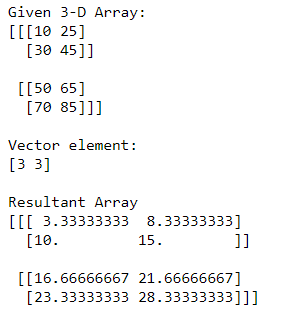GeeksforGeeks App
Open AppBrowser
Continue

# Divide each row by a vector element using NumPy

The following article depicts how to Divide each row by a vector element using NumPy. The vector element can be a single element, multiple element, or array. The division operator ( / ) is employed to produce the required functionality.  We can divide rows of 1-D, 2-D, or even more types of arrays with vector elements and the following examples will help you understand better:

### Divide row by a vector element in a 1-D Numpy array

In the example, we divide the rows of a 1-D Numpy array with a vector element i.e 

## Python3

 `# Importing Numpy module``import` `numpy as np` `# Creating 1-D Numpy array``n_arr ``=` `np.array([``20``, ``30``, ``40``])``print``(``"Given 1-D Array:"``)``print``(n_arr)` `# Vector element``vec ``=` `np.array([``12``])``print``(``"\nVector element:"``)``print``(vec)` `# Dividing rows of 1-D array with vector element``print``(``"\nResultant Array"``)``print``(n_arr ``/` `vec[:,``None``])`

Output:### Divide each by a vector element in a 2-D Numpy array

In the example, we divide each row by a vector element of a 2-D Numpy array with a vector element i.e [2.5]

## Python3

 `# Importing Numpy module``import` `numpy as np` `# Creating 2-D Numpy array``n_arr ``=` `np.array([[``20``, ``35``, ``40``],``                ``[``10``, ``51``, ``25``]])` `print``(``"Given 2-D Array:"``)``print``(n_arr)` `# Vector element``vec ``=` `np.array([``2.5``])``print``(``"\nVector element:"``)``print``(vec)` `# Dividing rows of 2-D array with vector element``print``(``"\nResultant Array"``)``print``(n_arr ``/` `vec[:,``None``])`

Output:### Divide row by a vector element in a 3-D Numpy array

In the example, we divide the rows of a 3-D Numpy array with a vector element i.e [3, 3]

## Python3

 `# Importing Numpy module``import` `numpy as np` `# Creating 3-D Numpy array``n_arr ``=` `np.array([[[``10``, ``25``], [``30``, ``45``]],``                  ``[[``50``, ``65``], [``70``, ``85``]]])` `print``(``"Given 3-D Array:"``)``print``(n_arr)` `# Vector element``vec ``=` `np.array([``3``, ``3``])``print``(``"\nVector element:"``)``print``(vec)` `# Dividing rows of 3-D array with vector element``print``(``"\nResultant Array"``)``print``(n_arr ``/` `vec[:,``None``])`

Output:My Personal Notes arrow_drop_up